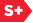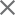# The world through a spinning window

Researchers revisit experiments begun over a century ago concerning the interplay between light and matter.
06 November 2007
Jonathan Leach, Joerg Goette, Les Allen, Miles Padgett, Amanda Wright, John Girkin, and Steve Barnett

In 1818, the French physicist Augustin-Jean Fresnel predicted that light passing through a moving medium would be dragged sideways, a phenomenon he called ether drag. Although the effect may not intrude on our everyday lives, it is of fundamental scientific interest. A century and a half later, R. V. Jones, an English physicist working at the University of Aberdeen in Scotland, set out to prove ether drag experimentally and to measure it for the first time.1,2

Jones showed that a beam of light falling normally (i.e., incident) on a block of glass moving with velocity v and length L was laterally displaced by a small amount, Δbeam, given bywhere n is the refractive index of glass and c is the speed of light. In addition to demonstrating the ether drag, Jones found that when transmitted along the rotation axis, the linear polarization state (the orientation of the electric field vector) was rotated through a small angle Δθpol3where Ω is the angular velocity or rotation rate of the medium. These experiments were supported by the theoretical analysis of M. A. Player4,5 and G. L. Rogers.6 The phenomenon has come to be known as the mechanical Faraday effect, owing to its similarities to Michael Faraday's finding in 1845 regarding the effect of a magnetic field on light.7

We became interested in the problem while working on the Abraham–Minkowski controversy, a 100-year-old debate about the momentum of light inside dielectric materials such as glass. But our focus was angular momentum as opposed to linear momentum, so we were concerned with rotation.

Due to the equivalence of spin and orbital angular momentum,8 the spinning medium should rotate not just the phase of a transmitted light beam but also the polarization state.9,10 The phase shift acquired by a transmitted light beam in fact manifests itself as an image rotation given byIn these phenomena, total displacement (see Equation 1) and total rotation (see Equation 3) represent the contribution from two separate terms. One is associated with the optical delay of light passing through a dielectric medium, Δdelay∝ n−1. The other has to do with refraction of the light in the rest frame—i.e., in which a particular object or system is not moving—of the medium, Δrefrac∝ 1−n−1 (see Figure 1). Note that in the rest frame the light is no longer at normal incidence but is inclined at an angle given by β = v/c.Figure 1. The transverse photon drag phenomenon as interpreted in both (a) the lab frame and (b) that of the moving medium. In the lab frame, the glass moves with a velocity v, and the light enters perpendicular to the surface. In the rest frame of the glass, the light moves with a velocity v and enters the glass at an angle β =v/c, where c is the speed of light.

Our initial approach to measuring this effect was to rotate a 20cm glass bar at approximately 200Hz using a geared system built on a washing machine motor. The predicted value for the image rotation is on the order of a few microradians. The noise in the current system, however, is larger than the predicted value, and we are currently working on alternative schemes.

The second approach was to switch to a case where we rotate or translate the light rather than the dielectric medium (see Figure 2). By interfering two light beams with a well-defined frequency shift, δ ν, between them, we can produce moving fringes—that is, the light patterns generated when two coherent beams combine—with a well-defined velocity. Alternatively, by interfering helically phased beams, the resulting pattern is that of a petal. If the beams differ in frequency, the petal spins with an angular velocity Ω=δν/2l, where l is the orbital angular momentum per photon in units of(Planck's constant). This second approach has the following advantage over the first: it is possible to frequency-shift light beams by tens of megahertz such that the angular velocity of the interference pattern is approximately 1 million orders of magnitude larger than that achieved with a rotating glass bar.Figure 2. Two interference patterns are generated and incident on a ground-glass screen. The screen is imaged onto the camera such that one of these interference patterns is captured through the 20cm-long glass bar, the other through free space.

This result raises an obvious question about whether the two cases—the first, where the glass is moving, and the second, where the image is moving—are equivalent, or whether there are subtle differences between them. It turns out that for rotating or translating images passed through a stationary dielectric medium, the measured rotation or displacement of the image scales as n−1, not as n−1/n as Equations 1 and 3 predict. Only Δdelay contributes to the measured effect. The Δrefrac term is not present.

When considering each frame of reference for this circumstance, it becomes apparent that the light always enters the medium at normal incidence. This is in contrast to the rotating or translating example, where the light is incident at an angle of β = v/c in the rest frame of the glass. In order to recover Δrefrac in the instance of the translating image, the medium must be tilted such that the angle of incidence is matched to β = v/c. Once this criterion is met, the measured delay is indeed the sum of Δdelay and Δrefrac (see Figure 3). Unfortunately, extension of the experiment to include the refractive terms is not so straightforward. The end faces of the glass bar would need to behave like an axicon (i.e., a cone), which is not possible given their flatness.Figure 3. The measured displacement of the nonlocalized interference fringes as a function of their translational velocity. The angle of the medium is adjusted to account for the angle of incidence of the light.
Conclusions

Beyond confirming the work of Jones, our experiments help to reveal the subtle relationships between light and moving media. However surprising it may seem, one consequence of these interactions is that the world viewed through a spinning window is indeed rotated.

Jonathan Leach, Joerg Goette, Les Allen, Miles Padgett
University of Glasgow
Glasgow, United Kingdom

Jonathan Leach is a research assistant at the University of Glasgow. Having completed his PhD on optical vortices, he is currently studying the effect of moving media on the properties of light.

Amanda Wright, John Girkin, Steve Barnett
University of Strathclyde
Glasgow, United Kingdom

Recent News